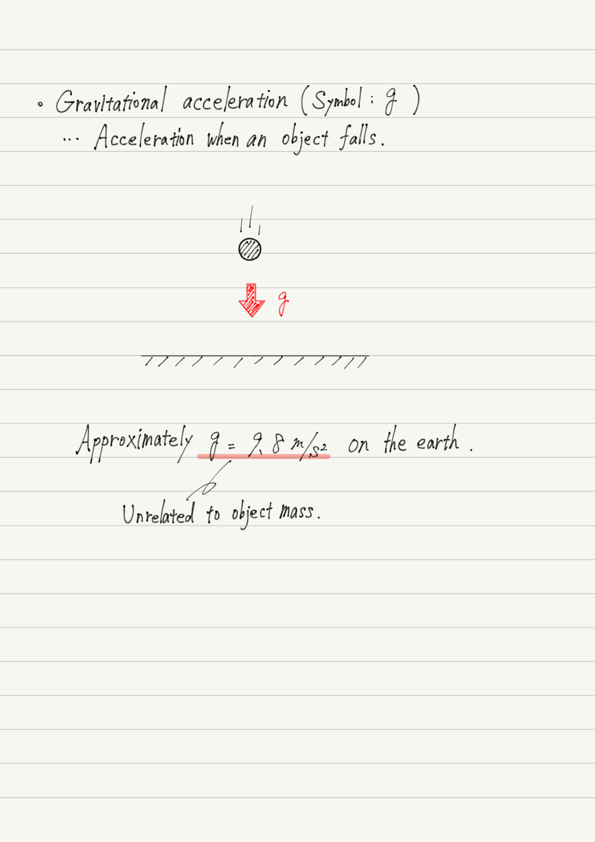# Gravitational Acceleration

Let’s learn about familiar uniform accelerated motion.

## Uniform accelerated motion around us

Uniform linear motion is ideal motion, not familiar motion, but what about uniform accelerated motion?

You may be thinking, “Isn’t it too convenient that the change in velocity is constant?”
In fact, however, uniform accelerated motion is a very familiar type of motion.

The most familiar example is falling objects. Everyone has dropped an object, and the object reaches the ground with gradually increasing velocity.
In other words, it is in an accelerated motion!

Although it is not possible to tell whether the motion is “iso-accelerative” by eye observation, a simple experiment can confirm that the falling motion is iso-accelerative.

## Acceleration of fall

Now, picture the falling motion (ignoring air resistance). Or actually drop something.

Dropping an object is a common phenomenon, so there is nothing unusual about dropping an object again after all this time.

But that is the point!

If no momentum is applied when an object is dropped, it will always fall the same way. The direction of fall is always directly down, and the acceleration is always the same.

The acceleration of a fall is thus a “special” acceleration, since it will be the same no matter when, who, where, or what is dropped.

This “special” acceleration is called gravitational acceleration. While acceleration is usually represented by the symbol a, gravitational acceleration is represented by g.

## Summary of this lectureGravitational acceleration is used very often not only in falling motion but also in physics in general, so it is important to keep it in mind.

## Next Time

In the next lecture, we will examine falling motion specifically.

https://www.yukimura-physics.com/entry/dyn-f08

error: Content is protected !!